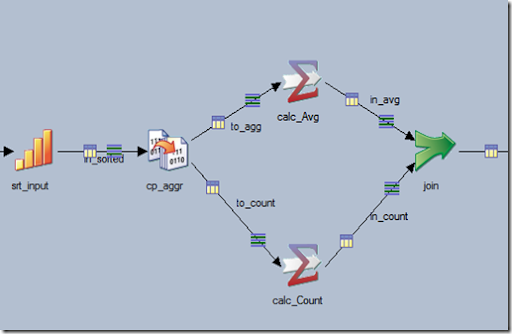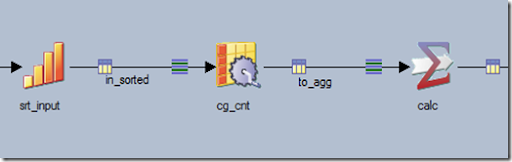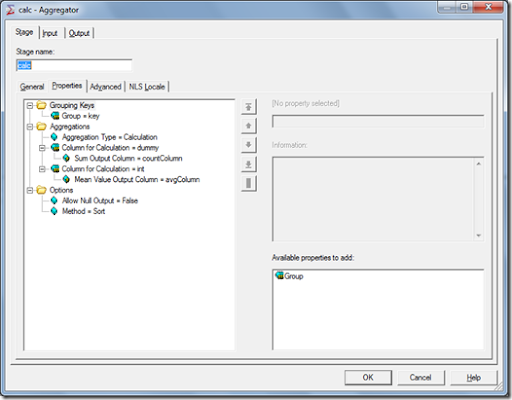## 05 August 2013

### DataStage Patterns – Calculate Count and Avg with the same Aggregator stage

Requirement: Build a job that calculates an average value for a group of rows and count the rows used in the calculation.

Solution: The DataStage Aggregator stage can either calculate an average value or count records, but not both at the same time. We could easily build a job like this:The first aggregator stage calculates the average and the second calculates the count of rows. The disadvantages are that we need to join the data back together and if the input is not sorted then a Hash aggregation should be forced (each stage will create its own hash table).

But what about if we want to do both calculations using a single aggregator stage? We can do it by introducing a new column (via column generator for example)  with constant value of “1”. Then we can sum this column using the same aggregator. The job will look like this: